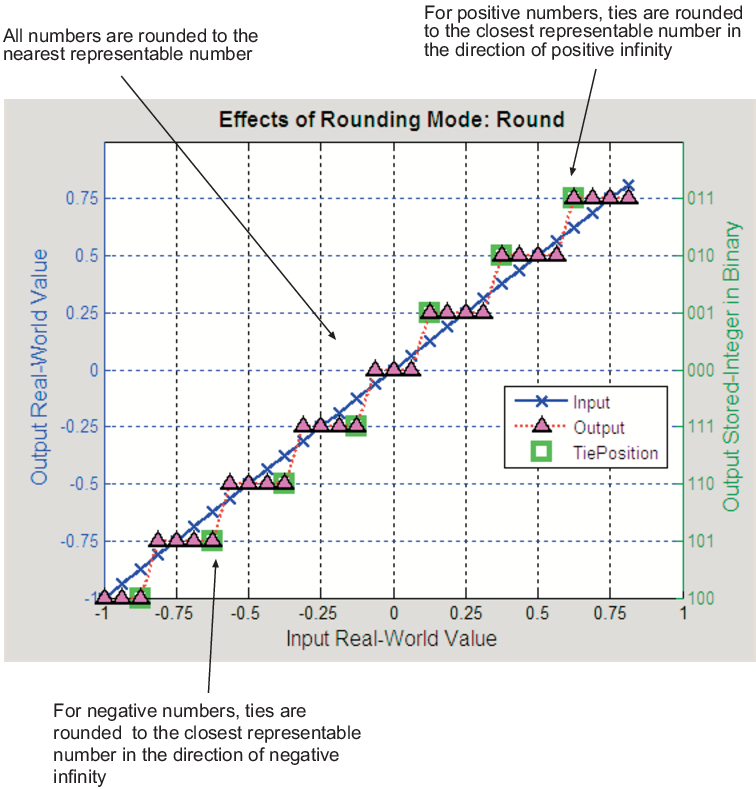## Rounding Mode: Round

`Round` rounds to the closest representable number. In the case of a tie, it rounds:

• Positive numbers to the closest representable number in the direction of positive infinity.

• Negative numbers to the closest representable number in the direction of negative infinity.

As a result:

• A small negative bias is introduced for negative samples.

• No bias is introduced for samples with evenly distributed positive and negative values.

• A small positive bias is introduced for positive samples.

In the MATLAB® software, you can perform this type of rounding using the `round` function. The rounding mode `Round` is shown in the following figure.##### SupportGet trial now